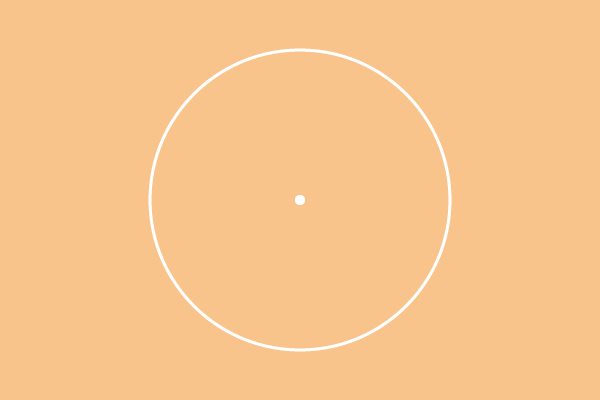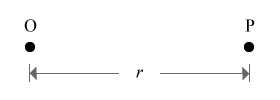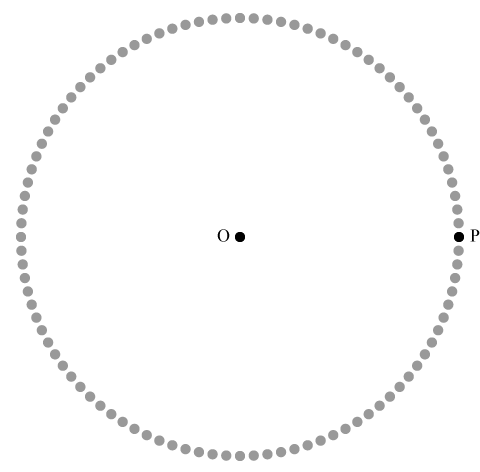# CircleThe locus of a moving point that maintains equidistant from a fixed point is defined as circle.

Circle is a plane round shape figure and it is one of the most significant geometrical shapes in geometry. It is actually formed in two different ways.

1. A point which moves around another point with equidistant.
2. Joining the points which are located at equal distance from a point.

These two methods form round shape figure geometrically and it is called as circle. Here, you understand how exactly a circle is formed in geometric system.

## ConstructionConsider a point in the plane and it is called as point O.

Consider another point in the plane and assume to call it as point P and it is located at r units’ distance from the point O.

Identify the points which are located at equal distance (r units) from the point O. Therefore, the distance from point O to point P is exactly equal to distance from point O to each surrounded point displayed in the below figure.Now, join all the points and it forms a round shape figure, called circle.

Instead, assume the point P is moved in round shape by maintaining equal distance from the point O. The point P is known as variable point because it represents all the points which are located at same distance from point O. The locus of the moving point gives a round plane figure and it is known as circle. The locus of variable point can be understood in this video tutorial.

A best free mathematics education website for students, teachers and researchers.

###### Maths Topics

Learn each topic of the mathematics easily with understandable proofs and visual animation graphics.

###### Maths Problems

Learn how to solve the math problems in different methods with understandable steps and worksheets on every concept for your practice.

Learn solutions

###### Subscribe us

You can get the latest updates from us by following to our official page of Math Doubts in one of your favourite social media sites.